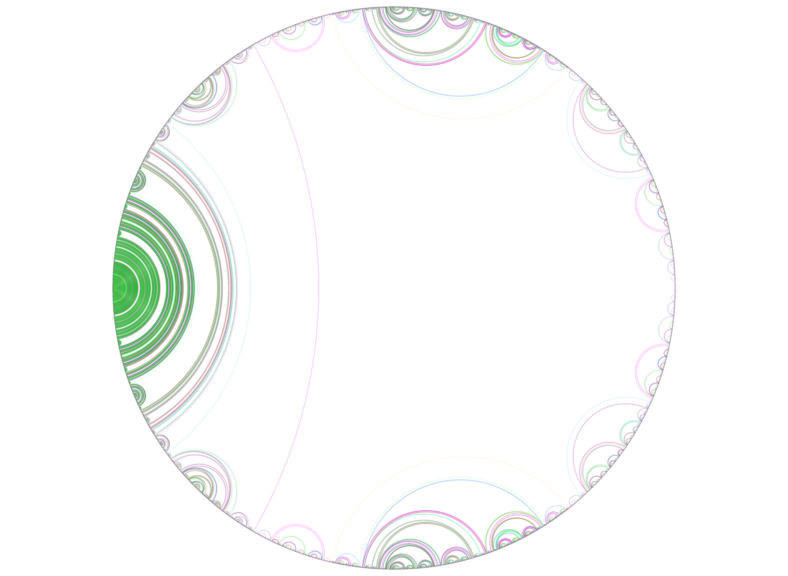# mathr / blog / #

## Lavaurs' AlgorithmI implemented Lavaurs' Algorithm to render an abstract Mandelbrot Set (in Haskell with SVG output via dirty string concatenation).

> import Data.Function (on)
> import Data.List (findIndex, groupBy)
> import Data.Ratio ((%))
> import Numeric (showHex)
> import System.Environment (getArgs)
>
> type N = Integer
> type Q = Rational

The doubling map identifies periods with points on the unit circle (as rational numbers where 1 is a full turn):

> double :: Q -> Q
> double p
>   | q >= 1 = q - 1
>   | otherwise = q
>   where q = 2 * p
>
> period :: Q -> N
> period p =
>   let Just i = (p ==) findIndex drop 1 (iterate double p)
>   in  fromIntegral i + 1

Generate an infinite list of rationals sorted by their period:

> denominators :: [(N, N)]
> denominators = iterate (\(p, d) -> (p + 1, 2 * d + 1)) (1, 1)
>
> rationals :: [Q]
> rationals =
>   [ q
>   | (p, d) <- denominators
>   , n <- [ 1 .. d - 1 ]
>   , let q = n % d
>   , period q == p
>   ]

The algorithm is implemented with a pair of mutually recursive functions. Initially, 1/3 and 2/3 are connected, then the next remaining rational is connected to the next smallest of the same period such that no lower-period arcs are intersected:

> lavaurs :: [(N, (Q, Q))]
> lavaurs = (2, (1%3, 2%3)) : lavaurs' (drop 2 rationals)
>
> lavaurs' :: [Q] -> [(N, (Q, Q))]
> lavaurs' (p : ps) =
>   let (qs, r : rs) = break (available p) ps
>   in  (period p, (p, r)) : lavaurs' (qs ++ rs)
> lavaurs' [] = error "broken invariant"
>
> available :: Q -> Q -> Bool
> available p q = null
>               . filter (intersects (p, q) . snd)
>               . takeWhile ((< period p) . fst)
>               $lavaurs > > intersects :: (Q, Q) -> (Q, Q) -> Bool > intersects (p, q) (s, t) > | q < s = False > | t < p = False > | p < s && t < q = False > | s < p && q < t = False > | otherwise = True The rest of the program is uninteresting code to output a pretty SVG image: > main :: IO () > main = do > [n] <- map read fmap getArgs > putStr header > putStr . concatMap g . take n . groupBy ((==) on fst)$ lavaurs
>   putStr footer
>   where
>       [ "<?xml version='1.0' encoding='UTF-8'?>"
>       , "<!DOCTYPE svg PUBLIC '-//W3C//DTD SVG 1.1//EN' 'http://www.w3.org/Graphics/SVG/1.1/DTD/svg11.dtd'>"
>       , "<svg xmlns='http://www.w3.org/2000/svg' width='4096' height='4096' viewbox='0 0 4096 4096'>"
>       , "<title>Mandelbrot Set by Lavaurs' Algorithm</title>"
>       , "<rect x='0' y='0' width='4096' height='4096' stroke='none' fill='white' />"
>       , "<g stroke-width='1' fill='none'>"
>       ]
>     footer = unlines
>       [ "</g>"
>       , "<circle cx='2048' cy='2048' r='2000' fill='none' stroke='black' stroke-width='1' />"
>       , "</svg>"
>       ]
>     g npqs = let (n:_, pqs) = unzip npqs
>              in  "  <g stroke='" ++ colours !! fromIntegral n ++ "'>\n" ++ concatMap arc pqs ++ "  </g>\n"
>     arc (p, q) = "    <path d='M" ++ px ++ "," ++ py ++ "A" ++ r ++ "," ++ r ++ " 0 0 1 " ++ qx ++ "," ++ qy ++ "' />\n"
>       where
>         pa = 2 * pi * fromRational p
>         qa = 2 * pi * fromRational q
>         r  = s $2000 * tan ((qa - pa) / 2) > px = s$ 2048 + 2000 * cos pa
>         py = s $2048 - 2000 * sin pa > qx = s$ 2048 + 2000 * cos qa
>         qy = s \$ 2048 - 2000 * sin qa
>         s :: Double -> String
>         s = show

With colours spread according to the Golden Angle:

> data RGB = RGB Double Double Double
>
> colours :: [String]
> colours = map (rgbToSVG . hueToRGB) [0, 2 * pi / (phi * phi) ..]
>   where
>     phi = (sqrt 5 + 1) / 2
>     hueToRGB h =
>       let s = 1
>           v = 1
>           hh = h * 3 / pi
>           i = floor hh mod 6 :: Int
>           f = hh - fromIntegral (floor hh :: Int)
>           p = v * (1 - s)
>           q = v * (1 - s * f)
>           t = v * (1 - s * (1 - f))
>       in  case i of
>             0 -> RGB v t p
>             1 -> RGB q v p
>             2 -> RGB p v t
>             3 -> RGB p q v
>             4 -> RGB t p v
>             _ -> RGB v p q
>
> rgbToSVG :: RGB -> String
> rgbToSVG (RGB r g b) =
>   let toInt x = let y = floor (256 * x) in 0 max y min 255 :: Int
>       hex2 i = (if i < 16 then ('0':) else id) (showHex i "")
>   in  '#' : concatMap (hex2 . toInt) [r,g,b]

References: